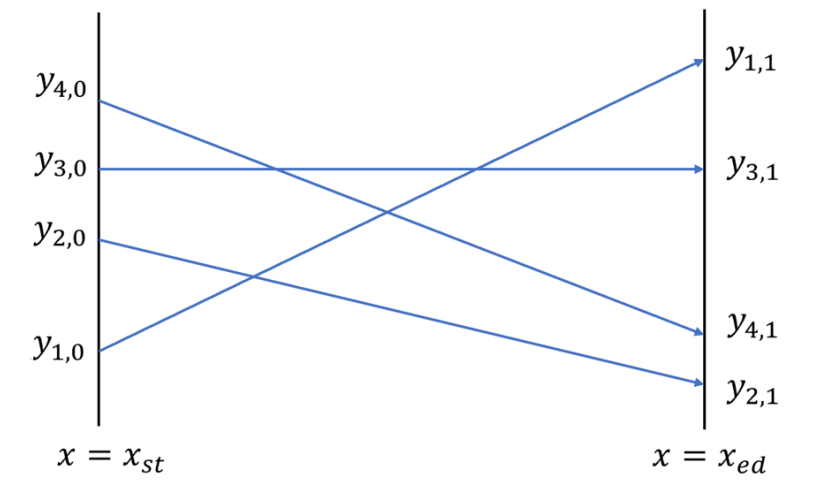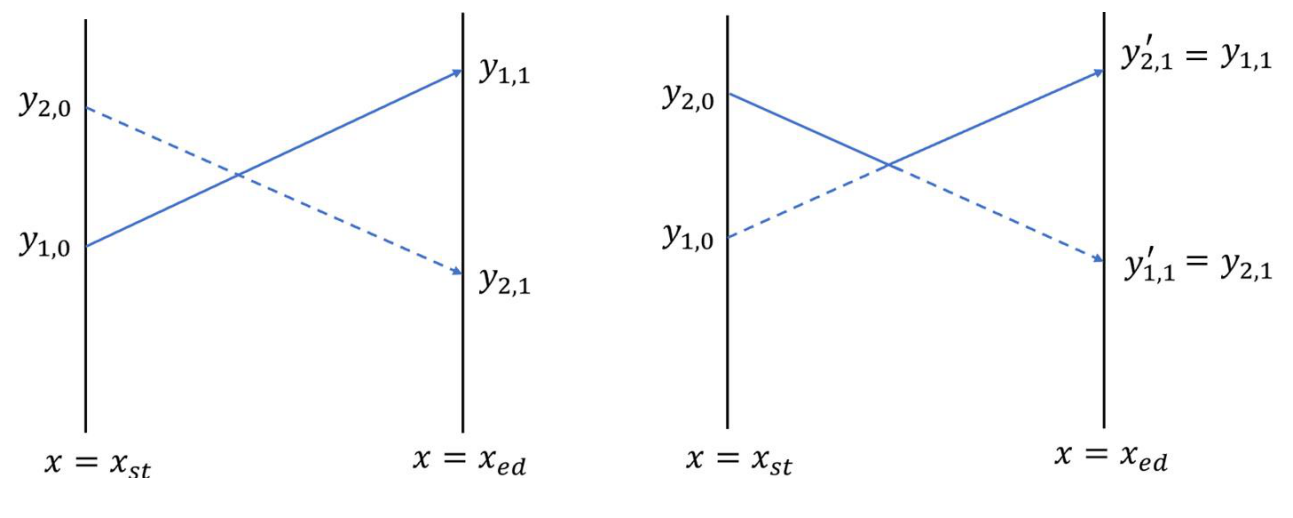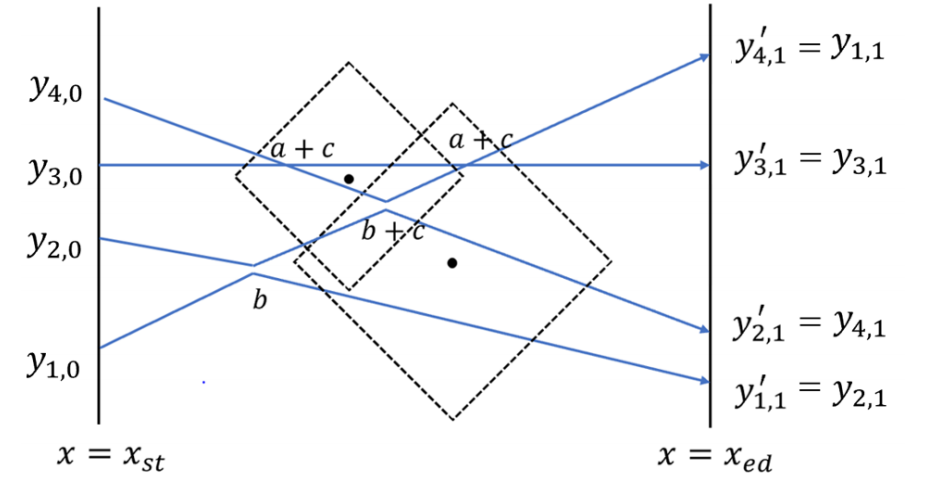TouchStone
请登录后使用
P5597[GXOI2019]特技飞行
 限制 : 时间限制 : - MS   空间限制 : - KB评测说明 : 2s,512m
###### 问题描述$n$ 架飞机同时出发且始终保持一定的对地速度。因此，对于任意两条交叉的航线（线段），对应的两架飞机必然会同时到达交点处——这就是它们进行特技表演的时刻。它们将会偏转机翼，展现以极近的距离「擦身而过」特技，然后继续保持各自的航线###### 样例输入 1

10 73 28 13 0 100
2 9 16 25 29 34 43 46 52 58
8 25 35 52 41 5 16 3 19 48
5
46 40 1
37 27 5
67 34 1
65 28 4
29 38 1

989 1619

4 1 2 3 1 6
1 2 3 4
4 1 3 2
2
3 3 1
5 2 2

13 15

###### 提示

$y_{i,0} < y_{i + 1,0}$$y_{i,1}$ 各不相同。
$x_{st} < p_i < x_{ed},1 ≤ a,b,c ≤ 10^3$

$1$ $n,k \le 15$ $\le 40$
$2$ $n,k \le 15$ $\le 40$
$3$ $n,k \le 15$ $\le 40$
$4$ $n,k \le 15$ $\le 40$
$5$ $n \le 30.000,k \le 100$ $\le 200,000$
$6$ $n \le 30.000,k \le 100$ $\le 200,000$
$7$ $n \le 30.000,k \le 100$ $\le 200,000$
$8$ $n \le 30.000,k \le 100$ $\le 200,000$
$9$ $n,k \le 100,000$ $\le 500,000$ $a = b$
$10$ $n,k \le 100,000$ $\le 500,000$ $a = b$
$11$ $n,k \le 100,000$ $\le 500,000$ $a = b$
$12$ $n,k \le 100,000$ $\le 500,000$ $a = b$
$13$ $n,k \le 50,000$ $\le 250,000$
$14$ $n,k \le 50,000$ $\le 250,000$
$15$ $n,k \le 50,000$ $\le 250,000$
$16$ $n,k \le 50,000$ $\le 250,000$
$17$ $n,k \le 100,000$ $\le 500,000$
$18$ $n,k \le 100,000$ $\le 500,000$
$19$ $n,k \le 100,000$ $\le 500,000$
$20$ $n,k \le 100,000$ $\le 500,000$